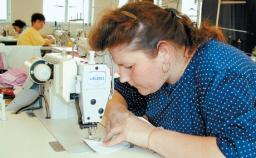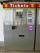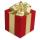# Seamstress

Seamstress bought two kinds of textile together 25 meters. One at 50 SKK and the second 70 SKK per meter. How many meter bought from this two textiles when paid totally 1540 SKK?

Correct result:

x =  10.5 m
y =  14.5 m

#### Solution:

50 x + 70 y = 1540
x+y = 25

50•x + 70•y = 1540
x+y = 25

50x+70y = 1540
x+y = 25

x = 212 = 10.5
y = 292 = 14.5

Our linear equations calculator calculates it.We would be pleased if you find an error in the word problem, spelling mistakes, or inaccuracies and send it to us. Thank you!Tips to related online calculators
Do you have a system of equations and looking for calculator system of linear equations?
Do you want to convert length units?

## Next similar math problems:

• Hotel roomsIn the 45 rooms, there were 169 guests, some rooms were three-bedrooms and some five-bedrooms. How many rooms were?
• Linear systemSolve this linear system (two linear equations with two unknowns): x+y =36 19x+22y=720
• EquationsSolve following system of equations: 6(x+7)+4(y-5)=12 2(x+y)-3(-2x+4y)=-44
• Tour35 people went on a tour and paid 8530, -. Employees pay 165, and family members 310. How many employees and how many family members went to the tour?
• TheatroTheatrical performance was attended by 480 spectators. Women were in the audience 40 more than men and children 60 less than half of adult spectators. How many men, women and children attended a theater performance?
• Three brothersThe three brothers have a total of 42 years. Jan is five years younger than Peter and Peter is 2 years younger than Michael. How many years has each of them?
• The dormitoryThe dormitory accommodates 150 pupils in 42 rooms, some of which are triple and some are quadruple. Determine how many rooms are triple and how many quadruples.
• Equations - simpleSolve system of linear equations: x-2y=6 3x+2y=4
• Two numbersWe have two numbers. Their sum is 140. One-fifth of the first number is equal to half the second number. Determine those unknown numbers.
• TreesAlong the road were planted 250 trees of two types. Cherry for 60 CZK apiece and apple 50 CZK apiece. The entire plantation cost 12,800 CZK. How many was cherries and apples?
• Stamps 2Dennis spent 34.15 on stamps. The number of .56 is 10 less than four times of stamps bought for .41. How many of each stamp did he buy?
• MushroomsEva and Jane collected 114 mushrooms together. Eve found twice as much as Jane. How many mushrooms found each of them?
• Waiting roomIn the waiting room are people and flies. Together they have 15 heads and 50 legs (fly has 6 legs). How many people and flies are in the waiting room?
• EurosPeter, Jane and Thomas have together € 550. Tomas has 20 euros more than Jane, Peter € 150 less than Thomas. Determine how much has each of them.
• CoinsIn ticket maschine were together one hundred coins. They were only 20 and 50 cent coins. The sum total was 29 euros and 60 cents. How many were in ticket maschine coins and which type?
• Money duoJulius and Mark have together 45 euros. Mark has 50% more money than Julius. Determine the amount of money that have Mark and Julius.
• JaneJane buy gift together for 464 Sk. For tie paid 4.5 times less than in the shirt, but 56 Sk more than for socks. How much she paid for each item in gift?